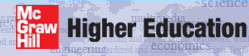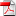# Institut für Thermodynamik der Luft- und Raumfahrt - Universität StuttgartMechanical Engineering - The University of Texas at AustinThe benchmark dataset is the flow over the suction side of a turbine vane at its design Reynolds number, documented experimentally by Daniels1 and numerically simulated by Daniels and Browne2 and Schmidt and Patankar3 and Rodi and Scheuerer4. This dataset belongs to the class of problems called the external flow, implying the solution requires the free stream velocity distribution as a boundary condition.

Turbine blading for gas turbines include the moving blades, generally called blades, and the stationary blades, generally called vanes. The blading geometry is generally airfoil in shape and subdivided into the pressure side and the suction side. While this section is specifically named turbine blade the procedures outlined in the section describing the construction of the dataset is equally applicable to all blading and to either the pressure or the suction side of the airfoil-shaped geometry.

Dataset Name - The turbine blade problem using the mixing length turbulence model is the benchmark dataset v700_5e.dat.txt.

note: While this data set has a designation "5e" which means it uses a mixing-length turbulence model and the Mayle transition model, the dataset contains extra variables for testing the one-equation and two-equation turbulence models. These extra variables can be thought of as placeholders for easily converting the dataset, because they are not read when neq=2, and they do not affect how TEXSTAN processes the mixing-length version of the dataset.

To create a two-equation turbulence model version of the dataset that uses the Lam-Bremhorst turbulence model with controlled transition, the major changes are to set neq=4, mode=2, ktmu=23, ktmtr=2, axx=125.0, and epsapp set either =8231.0 (specific for Daniels and Browne) or set =0.0, which causes TEXSTAN to compute an epsapp. With these changes the dataset becomes v700_23p.dat.txt.

Problem Description - The benchmark turbine blade flow occurs over the suction side of the airfoil with constant wall temperature and variable free stream velocity. The flow is a transitional boundary layer flow that remains attached from the stagnation point region to the blade trailing edge. The problem permits comparison of the predicted heat transfer coefficient distribution (W/m2-K) with experimental data. The problem description includes:

• u(x) - free stream velocity distribution (data from experimental measurements by Daniels)
• Tstag = 432K (the flow stagnation temperature, constant)
• Pstag = 290,000 Pa (the flow stagnation pressure, constant)
• Ts = 289K (the surface temperature, constant)
• Tu = 3 percent (estimated free stream u-component turbulence level)
• Vapp = 146.4 m/s (the approach velocity)
• fluid:
• air
• properties variable
note: this problem requires viscous dissipation but no body force effects are consideration
• Pr=0.9 (constant)
• turbulence model:
• mixing length model (ktmu=5)
• Mayle transition model (ktmtr=5)
note: this data set is easily converted to permit use of either a 1-equation or a 2-equation model of turbulence
• geometry:
• suction side surface length: 0.0643 meters
• note: overall flow length compares to a trailing edge x-Reynolds number of about 1.7E+06
• start condition: stagnation point flow (kstart=7) at an x-Reynolds number ~ 125
note: TEXSTAN may not start precisely at the stagnation point due to the coordinate transformations
• end condition: trailing edge (0.0643 meters)

## References

1
Daniels, L. D., "Film-Cooling of Gas Turbine Blades," Ph.D. Thesis, Dept. of Eng. Sci., U. of Oxford, 1978
2
Daniels, L. D., and Browne, W. B., "Calculation of Heat Transfer Rates of Gas Turbine Blades," Int. J. Heat Mass Transfer, Vol. 24, No. 5, pp. 871-879, 1981
3
Schmidt, R. C., and Patankar, S. V., "Simulating Boundary Layer Transition with Low-Reynolds Number k-e Turbulence Models: Part 2 - An Approach to Improving the Predictions," J of Turbomachinery, Vol. 113, pp. 18-26, Jan 1991